﻿ 干湿循环下岩石力学特性与能量演化规律的实验研究

# 干湿循环下岩石力学特性与能量演化规律的实验研究Experimental Research on the Mechanical Properties and Energy Evolution of Rock under Dry-Wet Cycle

Abstract: Underground water reservoir, hydroelectric power generation, basic engineering, sponge city construction, etc. will encounter the dry and wet cycling environment of rock. However, the process of dry wet cycle can degrade the rock. Therefore, it is of great scientific and engineering significance to study the law of energy evolution and its correlation mechanism of rock under the dry wet cycle for the deep understanding of rock deformation and fracture phenomenon under the dry wet cycle and the scientific decision-making of rock engineering construction and protection. In this paper, granite is selected as the research object. After 0 (without dry and wet cycle treatment), 1, 3, 6 and 10 times of dry and wet cycle, the uniaxial compression and energy evolution tests of rock samples after treatment are carried out, and the mechanical properties of granite after wet dry cycle are summarized combining with the characteristic parameters of acoustic emission.

1. 引言

2. 干湿循环试验

2.1. 岩样制备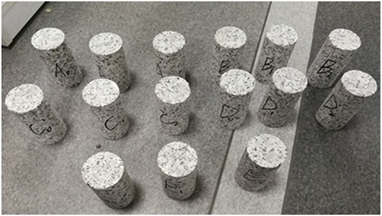Figure 1. Granite samples without dry and wet cycleTable 1. Initial size of granite sample

2.2. 干湿循环处理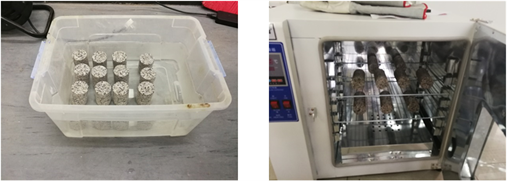Figure 2. Natural soaking and blast drying of graniteTable 2. Quality measurement results of granite samples after different dry and wet cycles

2.3. 纵波波速的测量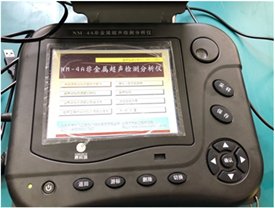Figure 3. NM-4 nonmetal ultrasonic testing analyzerTable 3. Measurement results of wave velocity of granite after different dry and wet cycles

3. 干湿循环后花岗岩能量演化规律

3.1. 单轴压缩试验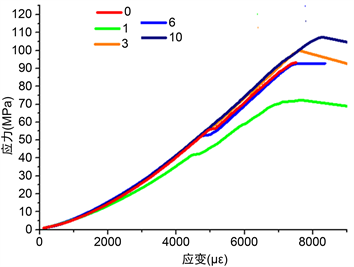Figure 4. The stress-strain curve of granite sample under conventional uniaxial compression test after different times of drying and wetting cycles

3.2. 单轴循环加卸载试验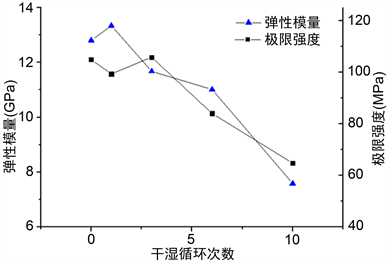Figure 5. The change of elastic modulus and ultimate strength of rock sample with the number of dry and wet cycles under uniaxial cyclic loading

3.3. 能量演化规律

$W={E}_{e}+{E}_{d}$ (1)

${u}_{id}=\underset{0}{\overset{{\epsilon }^{\prime }}{\int }}{\sigma }_{i}\text{d}{\epsilon }_{i}-\underset{{\epsilon }^{″}}{\overset{{\epsilon }^{\prime }}{\int }}{\sigma }_{i}\text{d}{\epsilon }_{i}$ (2)

${u}_{id}=\underset{{\epsilon }^{″}}{\overset{{\epsilon }^{\prime }}{\int }}{\sigma }_{i}\text{d}{\epsilon }_{i}$ (3)Figure 6. i-direction deviator stress deviator strain curve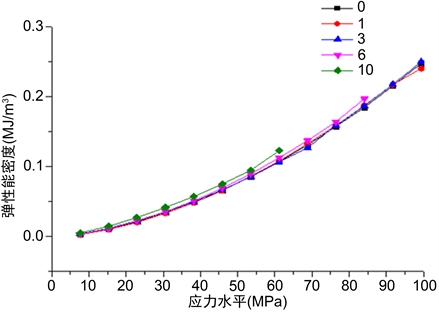Figure 7. Change of elastic energy density with stress level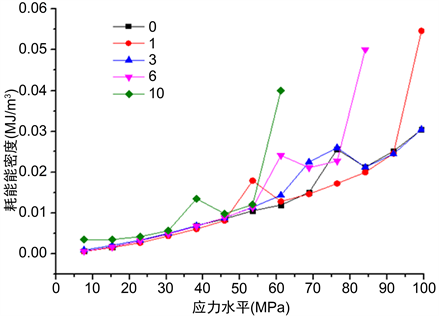Figure 8. Change of dissipative energy density with stress level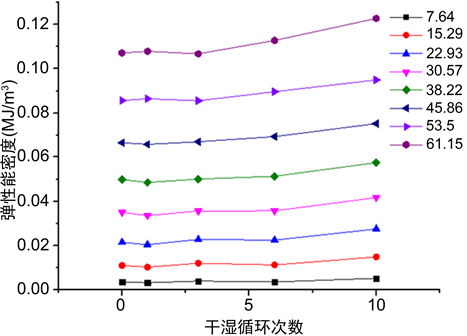Figure 9. The change of elastic energy density with the number of dry and wet cycles under different stress levels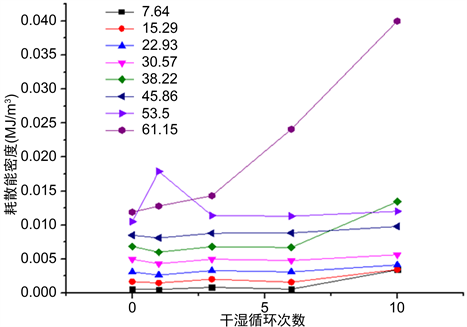Figure 10. The change of dissipative energy density with the number of dry and wet cycles under different stress levels

$U=\frac{\sigma \epsilon }{2}=\frac{{\sigma }^{2}}{2E}$ (4)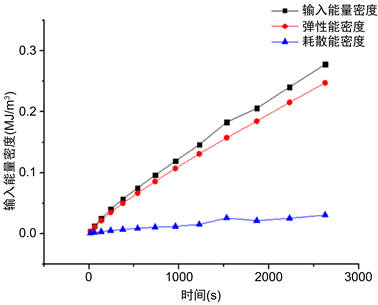Figure 11. Internal energy evolution of rock samples after 0 dry wet cycles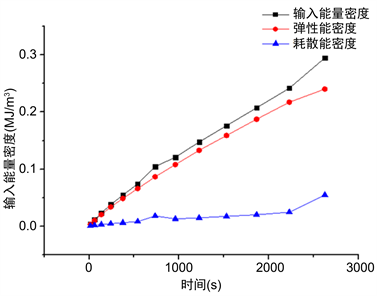Figure 12. Internal energy evolution of rock samples after 1 dry wet cycles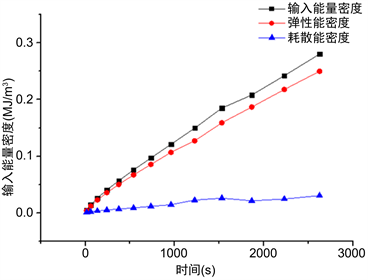Figure 13. Internal energy evolution of rock samples after 3 dry wet cycles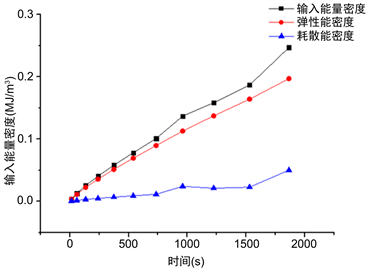Figure 14. Internal energy evolution of rock samples after 6 dry wet cycles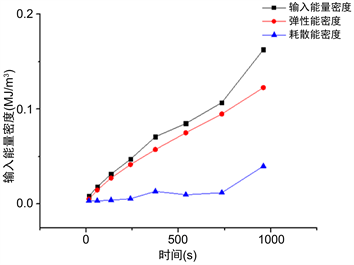Figure 15. Internal energy evolution of rock samples after 10 dry wet cycles

4. 干湿循环后花岗岩的声发射特征

4.1. 声发射信号特征参数

4.2. 声发射特征分析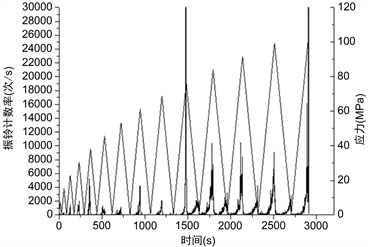Figure 16. The change of stress and ring count rate with time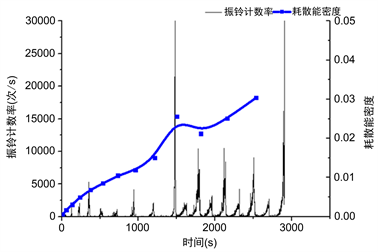Figure 17. Change of acoustic emission ringing rate and dissipative energy density of rock samples with time after 0 dry wet cycles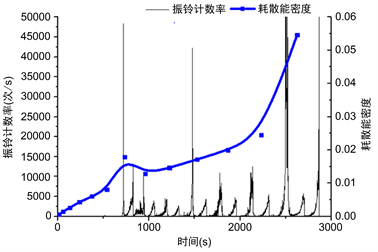Figure 18. Change of acoustic emission ringing rate and dissipative energy density of rock samples with time after 1 dry wet cycles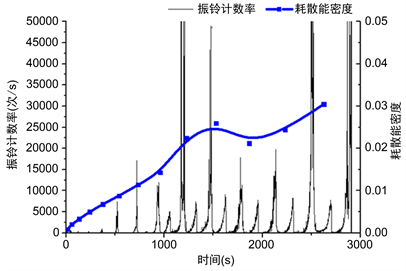Figure 19. Change of acoustic emission ringing rate and dissipative energy density of rock samples with time after 3 dry wet cycles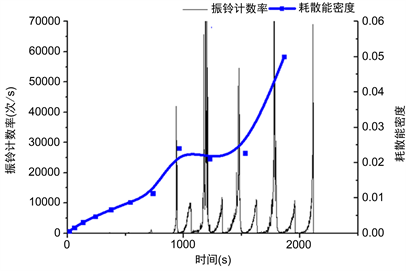Figure 20. Change of acoustic emission ringing rate and dissipative energy density of rock samples with time after 6 dry wet cycles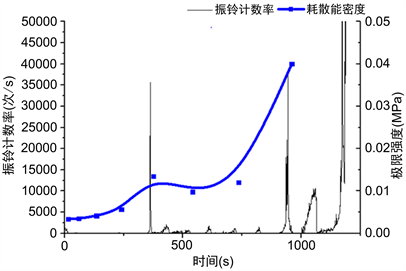Figure 21. Change of acoustic emission ringing rate and dissipative energy density of rock samples with time after 10 dry wet cycles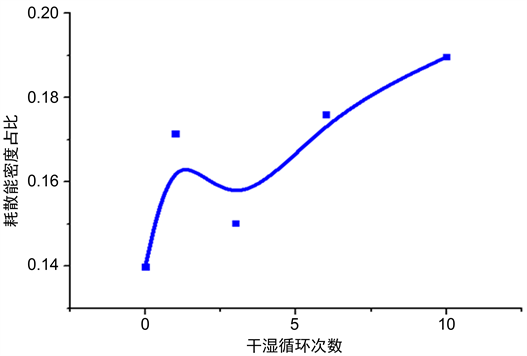Figure 22. Proportion of dissipated energy density at the first large peak of acoustic emission event event

5. 结论

1) 干湿循环条件对花岗岩有劣化作用：干湿循环后岩样质量和纵波波速变化不明显，但随着干湿循环次数的增加，花岗岩试样的强度极限和弹性模量将会降低。相同应力条件下，应变增大，弹性能增大，而耗散能增大更为剧烈。耗散能出现极大值时的时间会提前，应力水平会明显下降，耗散能密度占比提高。

2) 花岗岩岩样内部弹性能的演化几乎不受干湿循环条件的影响，花岗岩岩样的耗散能的演化会随着干湿循环次数的增加而明显增加，并且随着应力水平的增大，耗散能的增长率也变大。因此，输入能量的演化形态和耗散能的演化形态相似。

NOTES

*通讯作者。

 陶振宇. 岩石力学的理论与实践[M]. 北京: 水利出版社, 1981.

 董学晟. 水工岩石力学[M]. 北京: 中国水利水电出版社, 2004.

 刘新荣, 傅晏, 郑颖人, 等. 水岩相互作用对岩石劣化的影响研究[J]. 地下空间与工程学报, 2012, 1(8): 77-82.

 王伟, 刘桃根, 吕军, 王如宾, 徐卫亚, 翦波. 水岩化学作用对砂岩力学特性影响的试验研究[J]. 岩石力学与工程学报, 2012, 31(S2): 3607-3617.

 傅晏, 刘新荣, 张永兴, 胡元鑫, 谢应坤. 水岩相互作用对砂岩单轴强度的影响研究[J]. 水文地质工程地质, 2009, 36(6): 54-58.

 邓华锋, 胡安龙, 李建林, 张小景, 胡玉, 常德龙, 朱敏. 水岩作用下砂岩劣化损伤统计本构模型[J]. 岩土力学, 2017, 38(3): 631-639.

 王伟, 龚传根, 朱鹏辉, 朱其志, 徐卫亚. 大理岩干湿循环力学特性试验研究[J]. 水利学报, 2017, 48(10): 1175-1184.

 曾志雄, 孔令伟, 田海, 李聚昭. 膨胀岩崩解特性的干湿循环效应与粒度熵表征[J]. 岩土力学, 2017, 38(7): 1983-1989.

 李亚, 余宏明, 李科, 李雄峰. 干湿循环作用下石膏岩劣化效应的试验研究[J]. 长江科学院院报, 2017, 34(3): 63-66.

 梁冰, 谭晓引, 姜利国, 焦波波. 冻–融及干–湿循环对泥质岩崩解特性影响的试验研究[J]. 岩土工程学报, 2016, 38(4): 705-711.

 Badger, C.W., Cummings, A.D. and Whitmore, R.L. (1956) The Disintegration of Shale. Journal of the Institute of Fuel, 29, 417-423.

 Prick, A. (1995) Dilatometrical Behaviour of Porous Calcareous Rock Samples Subjected to Freeze Thaw Cycles. Catena, 25, 7-20.
https://doi.org/10.1016/0341-8162(94)00038-G

 Ojo, O. (1990) The Effect of Moisture on Some Mechanical Properties of Rock. Mining Science and Technology, 10, 145-156.
https://doi.org/10.1016/0167-9031(90)90158-O

 Obert, L., Windes, S.L. and Duvall, W.I. (1946) Standardized Tests for Determining the Physical Properties of Mine Rock. RI-3891, Bureau of Mines, U.S. Dept. of the Interior.

 宣以琼, 武强, 杨本水. 岩石的风化损伤特征与缩小防护煤柱开采机制研究[J]. 岩石力学与工程学报, 2005, 24(11): 1911-1916.

 Pardini, G., Guidi, G.V., Pini, R., Regues, D. and Gallart, F. (1996) Structure and Porosity of Smectite Mudrocks as Affected by Experimental Wetting-Drying Cycles and Freezing-Thawing Cycles. Catena, 27, 149-165.
https://doi.org/10.1016/0341-8162(96)00024-0

 Gökceoğlu, C., Ulusay, R. and Sönmez, H. (2000) Factors Affecting the Durability of Selected Weak and Clay-Bearing Rocks from Turkey, with Particular Emphasis on the Influence of the Number of Drying and Wetting Cycles. Engineering Geology, 57, 215-237.
https://doi.org/10.1016/S0013-7952(00)00031-4

 张志镇, 高峰. 受载岩石能量演化的围压效应研究[J]. 岩石力学与工程学报, 2015, 34(1): 1-11.

 张志镇, 高峰. 单轴压缩下岩石能量演化的非线性特性研究[J]. 岩石力学与工程学报, 2012, 31(6): 1198-1207.

 张志镇, 高峰. 单轴压缩下红砂岩能量演化试验研究[J]. 岩石力学与工程学报, 2012, 31(5): 953-962.

 张志镇. 岩石变形破坏过程中的能量演化机制[D]: [博士学位论文]. 徐州: 中国矿业大学, 2013.

Top